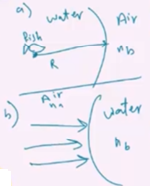Need Help?

Subscribe to Physics 2

###### \${selected_topic_name}
• Notes

$\begin{array}{l}{\text { A person is lying on a diving board } 3.00 \mathrm{m} \text { above the sur- }} \\ {\text { face of the water in a swimming pool. The person looks at a penny }} \\ {\text { that is on the bottom of the pool directly below her. The penny }} \\ {\text { appears to the person to be a distance of } 8.00 \mathrm{m} \text { from her. What is }} \\ {\text { the depth of the water at this point? }}\end{array}$

$s^{\prime}=8-3=5 m$

$S=? ?$

$R=\infty$

$\frac{n a}{s}+\frac{n b}{s^{\prime}}=0$

$n_{a}=1.33$

$n p=1$

$\frac{n_{a}}{S} =\frac{-n_ b}{s'}$

$∴ S=\frac{-n a}{n b} {S'}$

$S=-\left(\frac{1.33}{1}\right) (-5) \simeq 6.66 m$

$\begin{array}{l}{\text { A Spherical Fish Bowl. A small tropical fish is at the }} \\ {\text { center of a water-filled, spherical fish bowl } 28.0 \mathrm{cm} \text { in diameter. }} \\ {\text { (a) Find the apparent position and magnification of the fish to an }} \\ {\text { observer outside the bowl. The effect of the thin walls of the bowl }} \\ {\text { may be ignored. (b) A friend advised the owner of the bowl to keep }} \\ {\text { it out of direct sunlight to avoid blinding the fish, which might }} \\ {\text { swim into the focal point of the parallel rays from the sun. Is the }} \\ {\text { focal point actually within the bowl? }}\end{array}$

$R=\frac{28}{2}=14 \mathrm{cm}$

$\frac{n^{a}}{s_{1}}+\frac{n b}{s'}=\frac{n b-n_{a}}{R}$

$\frac{1.333}{14}+\frac{1}{s'}=\frac{1-1.333}{-14}$

$∴ s'=-14 cm$

$m=\frac{-n a s'}{n b s}$

$=\frac{-(1.333)(-14)}{1 \times 14}=1.33$

$S=\infty$

$n a=1 \quad, n b=1.333$

$R=+14 cm$

$\frac{n b}{s^{\prime}}=\frac{n_{b} n_ a}{R}$

$\frac{1.333}{s'}=\frac{1.333-1}{14}$

$∴ {s'}=+56 \mathrm{cm}$